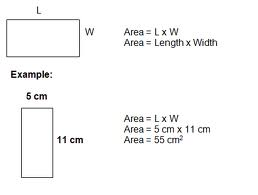HOW DO YOU CALCULATE THE VOLUME OF A RECTANGLE?

0  Views: 721 Answers: 2 Posted: 8 years ago

Width X Length = Square feet.

Width X Length X Height = Cubic feet.

I believe volume is generally calculated by cubic feet in that instance, unless specifically requested otherwise.

Rectangle doensn't have volume, it is a two dimensional polygon. Rectangle's aera is calculated by multiplying two adjacent sides' lengths with each other, as can be seen in the following picture:Calculation of a box's volume: multiplying the 3 sides' lengths with one another, as can be seen in the following picture:### Top contributors in Mathematics category

ROMOS
Karma: 13510

Colleen
Karma: 6890

country bumpkin
Karma: 6240

Bob/PKB